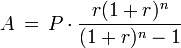# Everyday Calculation

Free calculators and unit converters for general and everyday use.

Calculators » Finance » Loan APR

## Loan Rate (APR) Calculator

Our online tools will provide quick answers to your calculation and conversion needs. On this page, you can calculate the annual percentage rate (APR) or the interest rate applicable on a mortgage loan given the loan amount and monthly payment.

Result window

Download: Use this loan calculator offline with our all-in-one calculator app for Android and iOS.

#### Advanced loan calculator

To calculate loan APR where payment is made in daily, quarterly or yearly installments, use the present value of annuity calculator. Select "Solve for Interest rate" and enter the loan amount in the present value field.

#### Loan APR formula

The calculation is arrived at by successively guessing a interest rate (between 0 and 100) and solving it for "payment amount" in the Amortization formula given below:Where,
P = principal amount borrowed,
A = periodic payment,
r = interest rate in % divided by 12,
n = total number of months